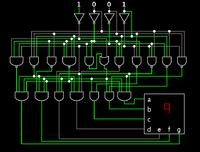# 3-digit 7-segment LED

Status
Not open for further replies.

#### Karali

##### Newbie level 1
Hello everybody,
I have a specific question to the following challenge:

There are seven resistors (R1, R2, ... , R7) which have one common node. Now I cut through those resistors depending on the desired binary code, e.g. when I cut the resistor R2 and R3 I get the following binary code: 0000110 with MSB = R7 and LSB = R1.

Now there are several solutions to read those values out. The simplest way is to measure the resistance of each resistor with respect to the common node with a multimeter. Since this is very impractical, I would like to contact those contact points all at once using eight contact spring probes and display the binary code as a decimal number using three 7-segment LEDs.

Now what would you recommend? I first started with a truth-table and then I wanted to simplify the problem using KV diagram until I noted that it is very extensive when you have seven input-variables... I already googelt and found out that when you want to use more than one 7-segment LED you have to mupliplex the whole circuit using a microcontroller, several logical building blocks, etc. and then you also have to program it.

Aren't there easier ways? e.g. only by using a 7-segment decoder and some logic gatters?!

Regards,
Karali

You need a way to convert binary numbers into BCD (binary coded decimal).

With BCD each decimal digit occupies 4 bits (a nibble). Each nibble goes up to 9. The next increment causes the BCD digit to return to 0, and generates a carry to the next nibble.

Say your resistors all transmit a '1'. In binary this occupies 2 nibbles as:
0111 1111

This number is decimal 127. In BCD this occupies 3 nibbles as:
0001 0010 0111

Each group would go to a 7 segment LED display.

You will need a very elaborate network of gates to make such a conversion.

Here is a schematic which will translate binary 0 to 9 to the 7-segment LED display.To expand this to 3 digits would be an enormous task.

Discussions at other forums frequently advise using a computerized chip (pic, etc.). If you want to avoid this, there is an IC called the 14553 '3-digit BCD counter'. It will count up to a number between decimal 0 and 999, and display it as 3 digits, multiplexed.

You would send a clock signal to its counter, as well as a binary counter, simultaneously. You would let both run until the binary counter matches your 7 resistors. The 14553 will have duplicated this same value encoded as BCD, and will display it on your 3 digit LED display.

Status
Not open for further replies.